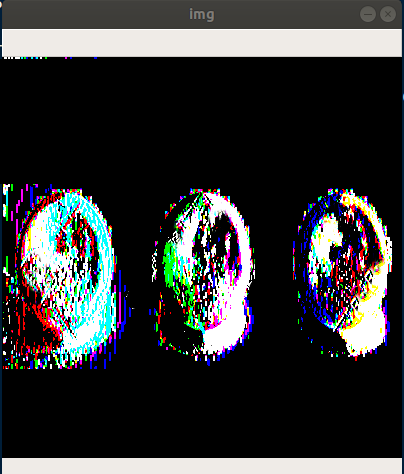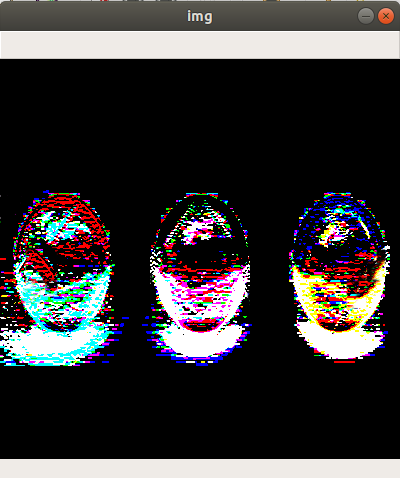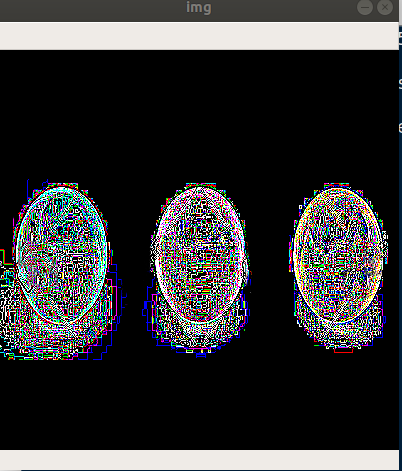## Edge Detection with Python using OpenCv

##### In this part of Learning Python we Cover Edge Detection Techniques In Python
Written by |10-May-2019 | 0 Comments | 293 Views

There are multiple ranges of edge detector which can be used in different scenarios. In this lecture we are going to see Sobel and Laplacian formula to find the edges:

Sobel Edge Detector:

Sobel edge detector uses the function of derivation to find the edges within the image. It is a gradient-based method and uses the first order derivative. The derivatives are calculated separately on the X axis and Y axis. The Sobel edge detector uses the kernel which iterates over the image as a window and transforms the image. The main thing that makes Sobel different from other edge detectors is that the kernel not only one rather than here the kernels are 2.

First, we will see how the Sobel for X-axis works. Moreover, the image we use for this example is 3 Balls of three colors: red, green, blue.Following is the code to implement the SobelX:

`import cv2`
`import numpy as np`

`img = cv2.imread('balls.jpg')`
`img = cv2.resize(img,(400,400))`
`SobelX = cv2.Sobel(img, cv2.CV_64F,1,0,5)`
`cv2.imshow("img",SobelX)`
`cv2.waitKey(0)`

The Sobel took five arguments. The first one is the object of an image. The second one is the flag of derivation. The third one and the fourth one, are the X or Y if X is one then Y is zero, and vice versa and the last one is the size of the kernel. It took only one parameter and made the height of kernel as of the width of the kernel.

The image that is generated as a result is as follows:Figure 1

If we have a close up of this image, we can see the pixel will form vertical lines which indicate that Sobel is implemented on the X-axis.

Similarly, we can do this on the y-axis as follows:

`import cv2`
`import numpy as np`

`img = cv2.imread('balls.jpg')`
`img = cv2.resize(img,(400,400))`
`SobelY = cv2.Sobel(img, cv2.CV_64F,0,1,5)`
`cv2.imshow("img",SobelY)`
`cv2.waitKey(0)`

Now the image will be the same, but the horizontal pixel lines form the edges lines. The image generated as a result is as follows:Figure 2

Laplacian Filter:

Unlike Sobel edge detector Laplacian edge detector uses only one kernel. Laplacian edge detector can find the second order derivative in one pass.

Following is the example of implementing the code:

`import cv2`
`import numpy as np`

`img = cv2.imread('balls.jpg')`
`img = cv2.resize(img,(400,400))`
`Laplacian = cv2.Laplacian(img, cv2.CV_64F)`
`cv2.imshow("img",Laplacian)`
`cv2.waitKey(0)`

Moreover, the image that is generated as a result is as follows:Figure 3

These are all the types of edge detectors that we can use with other filters to get our desired results. These filter sometimes need tuning, and some of these filters need to be combined with another filter to increase efficiency. It depends on the type of image and the type of task that you are going to perform on that particular image.

Now we will see what the threshold of an image is. How to convert an image into threshold image and what are the different types of threshold that we can use.

Threshold:

So, the first question what is the threshold? Thus, the answer to this question is that it is the technique to make everything either black or white. Suppose we have a threshold value of 150 than any pixel less than 150 is black and any pixel greater than 150 will be white. Then after applying a threshold, there will be no grey part of the image it will be either black or white. Now the OpenCV provides different type of threshold. We will see one by one the different kinds of threshold:

Simple Thresholding:

This method is straight forward. If the image pixel is higher than the specific value, the pixel is assigned the value of white pixel which is 255 and if the pixel value is less than defined value than it will assign the value of the black pixel that is 0. It took four arguments; at first, it took the object of an image. It is to be noted that the image should be grayscaled; otherwise, it will generate an error. The second argument is a minimum value at which the pixel should be differentiated whether it is a white pixel or black pixel. The third one is the maximum value. Moreover, the fourth one is the flag by which it will differentiate which threshold is to be applied.

Now we will see how we can implement the code. The image that we are going to use or this purpose is as follows:Figure 4

Figure 4 is the same document which we have written above. We make it flip left so that it can be identified as an image.

Thresh Binary:

The first threshold we are going to use thresh binary. This threshold changes the image into 0 or 1 means either in black or white.

`import cv2`
`import numpy as np`

`img = cv2.imread('doc.png',0)`
`_, thresh = cv2.threshold(img, 127, 255, cv2.THRESH_BINARY)`
`cv2.imshow("img", thresh)`
`cv2.waitKey(0)`

The image that is generated as a result can be seen in Figure 5:Figure 5

You have seen the above image. It is too much clear because there was no noise in an image previously.

Thresh Binary Inverse:

It is the opposite of the above method it will make the black pixel white and white pixel black. Following is the code to implement this method.:

`import cv2`
`import numpy as np`

`img = cv2.imread('doc.png',0)`
`_, thresh = cv2.threshold(img, 127, 255, cv2.THRESH_BINARY_INV)`
`cv2.imshow("img", thresh)`
`cv2.waitKey(0)`

The image generated as a result is as follows:Figure 6

The image pixel gets inverted in this method.

Thresh Trunc:

In this method, the white pixel, are converted into gray. Following is the code to implement this method:

`import cv2`
`import numpy as np`

`img = cv2.imread('doc.png',0)`
`_, thresh = cv2.threshold(img, 127, 255, cv2.THRESH_TRUNC)`
`cv2.imshow("img", thresh)`
`cv2.waitKey(0)`

The image that is generated as a result is the following:Figure 7

In the above image, the white pixels are manipulated and even the black pixel become lighter. These threshold effects make the white pixels in a range from black to white.